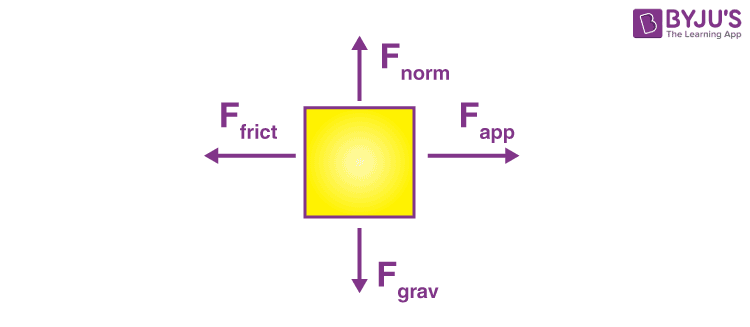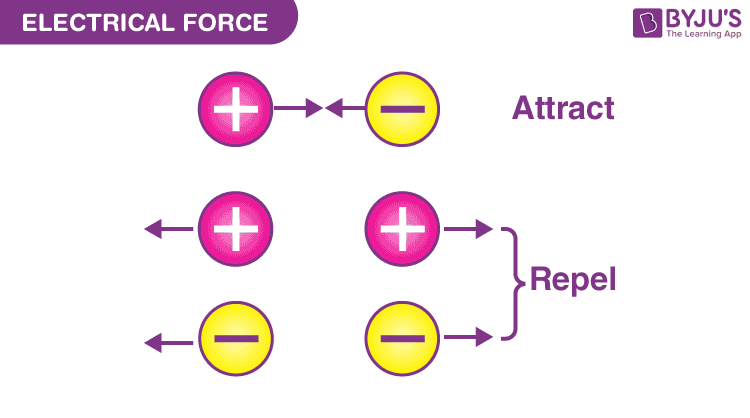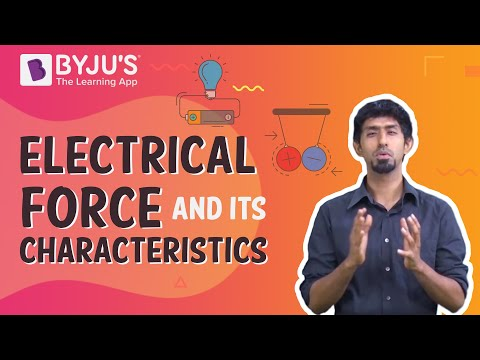# Electrical Force

## How Would You Define an Electrical Force?

The repulsive or attractive interaction between any two charged bodies is called as electric force. Similar to any force, its impact and effects on the given body are described by Newton’s laws of motion. The electric force is one of the various forces that act on objects.

Newton’s laws are applicable to analyse the motion under the influence of that kind of force or combination of forces. The analysis begins by the construction of a free body image wherein the direction and type of the individual forces are shown by the vector to calculate the resultant sum, which is called the net force that can be applied to determine the body’s acceleration.## What Does the Size of the Electric Force Depend On?

The electric force between two electrons is equal to the electric force between two protons when placed at equal distances. This describes that the electric force is not based on the mass of the object but depends on the quantity known as the electric charge.## What Is Coulomb’s Law?

Coulomb’s law is an experimental law that quantifies the amount of force between two stationary electrically charged particles. The electric force between a stationary charged body is conventionally known as the electrostatic force or Coulomb’s force. Coulomb’s law describes the amount of electrostatic force between stationary charges.

Coulomb’s law states that:

The value of the electrostatic force of interaction between two point charges is directly proportional to the scalar multiplication of the charges and inversely proportional to the square of the distance among them.

### What Is the Formula of Electric Force?

Electric force formula can be obtained from Coulomb’s law as follows:

 $$\begin{array}{l}\vec{F}=K\frac{q_{0}q_{1}}{r^{2}}\hat{r}\end{array}$$

Where,

• $$\begin{array}{l}\vec{F}\,\, is \,\,the \,\,electric \,\,force \,\,directed\,\, between\,\, two \,\,charged\,\, bodies.\end{array}$$
• K is the constant of proportionality.
• q0 and q1 are the amounts of charge on each body.
• r is the distance between the charged bodies.
• $$\begin{array}{l}\hat{r}\,\, is\,\, the\,\, variable\,\, unit\,\, vector\end{array}$$

## Watch the video and learn about the characteristics of electrical force## Electrical Force Examples

The examples of electric force are as mentioned below:

• The charge in a bulb.
• Electric circuits.
• Static friction between cloth when rubbed by a dryer.
• The shock that is felt after touching a doorknob.

The electric force can also be viewed through current electricity, like copper wiring that carries power to the whole building. The electrostatic force exhibits electric energy through static charges like cathode-ray tubes in TVs and electrostatic spray painting.

## Frequently Asked Questions – FAQs

Q1

### What is the unit of electrical force?

The electrical force, like other forces, is generally measured in Newton units.
Q2

### What is the name for an electric force?

Because of their electric charge, particles or objects are attracted to or repelled by the electrostatic force is also known as “Coulomb Force”.
Q3

### What are the types of electric force?

Electrical forces may be divided into two categories: attractive electrical forces and repulsive electrical forces. Similar charges repel one another, whereas opposite charges attract one another.
Q4

### What do electrical forces depend on?

Electric force strength is determined by the electric charge on the particles, as well as their separation from one another. The force increases with larger charges or closer distances.
Q5

### Why is electric force stronger than gravitational?

This is because gravity is mass-dependent, and because atoms have such little masses, the gravitational forces between them are near zero. However, the electrostatic force is associated with charges.

Stay tuned with BYJU’S to know more about magnetic force examples, along with Coulomb’s law examples.

Test Your Knowledge On Electrical Force!